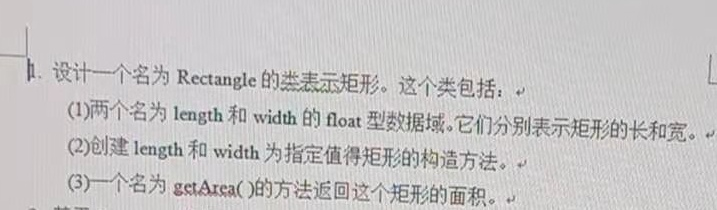2021-06-26 09:47

# 设计一个名为 Rectangle的类表示矩形• 写回答
• 好问题 提建议
• 关注问题
• 收藏
• 邀请回答

#### 2条回答默认 最新

•已采纳
``````#include<bits/stdc++.h>
#include<math.h>
using namespace std;
class Rectangle
{
private:
float length;
float width;
public:
void Set(float l,float w)
{
length=l;
width=w;
}
void getArea()
{
float s;
s=length*width;
cout<<"该长方形的面积为："<<s<<endl;
}
};
int main()
{
Rectangle r1;
float l,w;
cin>>l>>w;
r1.Set(l,w);
r1.getArea();

}
``````已采纳该答案
评论
解决 无用
打赏 举报
•``````class Rectangle
{
private:
float length;
float width;
public:
Rectangle() {}
Rectangle(float l,float w) {length = l;width = w;}
float getArea() {return length * width;}
};

int main()
{
Rectangle r(10.0,20.0);
float area = r.getArea();
cout<<"矩形面积为:"<<area<<endl;
return 0;
}
``````
评论
解决 无用
打赏 举报